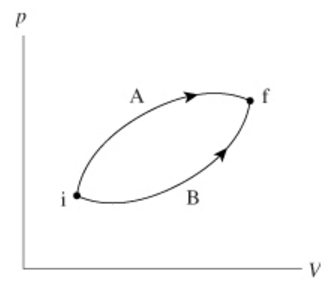# Problem: The figure (Figure 1) shows two different processes taking an ideal gas from state i to state f.Part A. Is the temperature change ΔT during process A larger than, smaller than, or equal to the change during process B?A. ΔTA &lt; ΔTB B. ΔTA = ΔTB C. ΔTA &gt; ΔTB Part B. Is the heat energy added during process A greater than, less than, or equal to the heat added during process B?A. QA = QB B. QA &gt; QB C. QA &lt; QB

###### FREE Expert Solution

Part A

It is seen from the figure that process A takes a higher volume than process B.

However, the change in temperature is the same for both the processes. This is because the change in internal energy is path independent.

The change in internal energy is only dependent on the initial and the final points.

96% (204 ratings)###### Problem Details

The figure (Figure 1) shows two different processes taking an ideal gas from state i to state f.Part A. Is the temperature change ΔT during process A larger than, smaller than, or equal to the change during process B?

A. ΔTA < ΔTB

B. ΔTA = ΔTB

C. ΔTA > ΔTB

Part B. Is the heat energy added during process A greater than, less than, or equal to the heat added during process B?

A. QA = QB

B. QA > QB

C. QA < QB